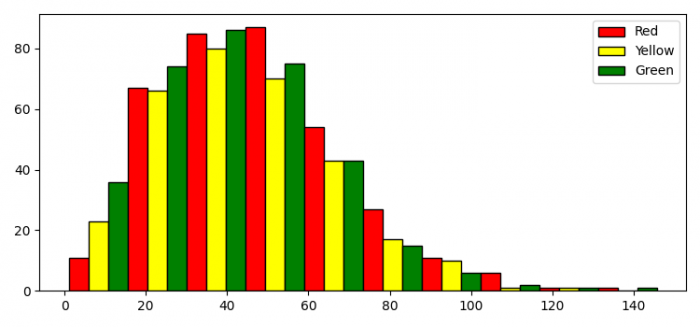# Matplotlib histogram with multiple legend entries

To plot a histogram with multiple legend entries, we can take the following steps −

• Set the figure size and adjust the padding between and around the subplots.
• Create random data using numpy
• Plot a histogram using hist() method.
• Make a list of colors to color the face of each patch.
• Iterate the patches and set face color of each patch.
• Create a list of handles to place the legend.
• Use legend() method for multiple legend entries.
• To display the figure, use show() method.

## Example

import matplotlib.pyplot as plt
import numpy as np
from matplotlib.patches import Rectangle

plt.rcParams["figure.figsize"] = [7.50, 3.50]
plt.rcParams["figure.autolayout"] = True

data = np.random.rayleigh(size=1000) * 35
N, bins, patches = plt.hist(data, 30, ec="k")
colors = ["red", "yellow", "green"]

for i in range(0, len(bins)-1):
patches[i].set_facecolor(colors[i % len(colors)])

handles = [Rectangle((0, 0), 1, 1, color=c, ec="k") for c in colors]
labels = ["Red", "Yellow", "Green"]
plt.legend(handles, labels)

plt.show()

## Output